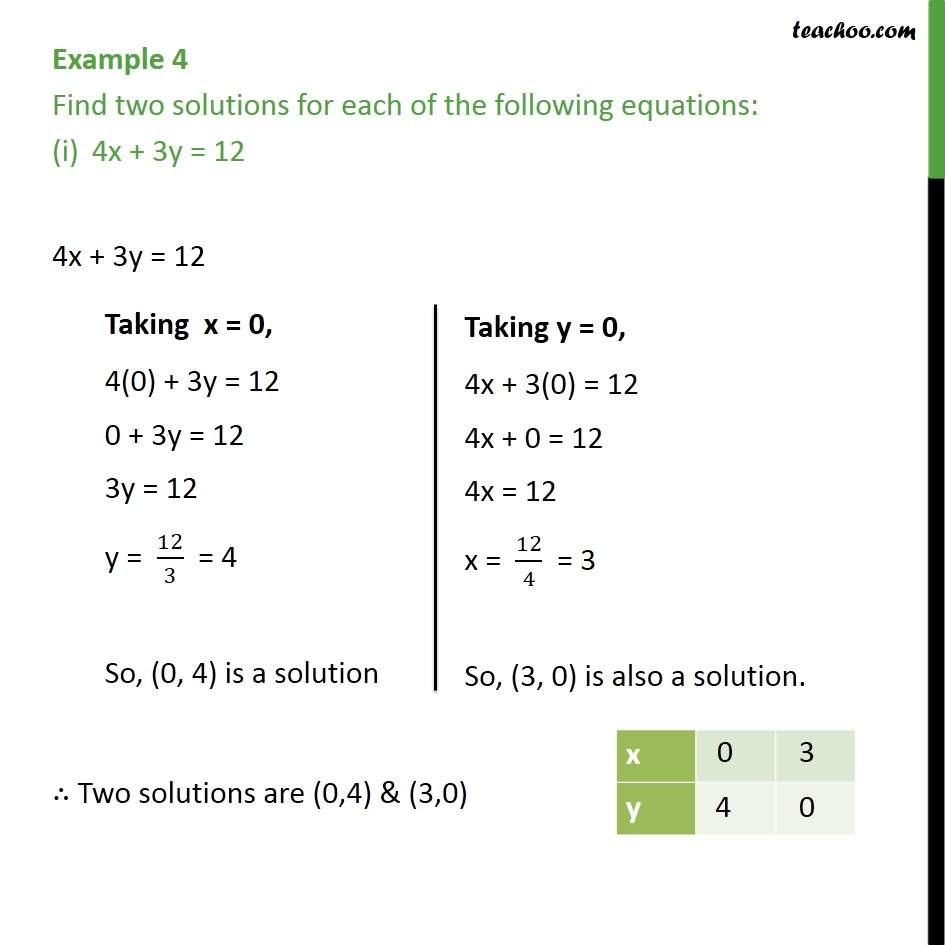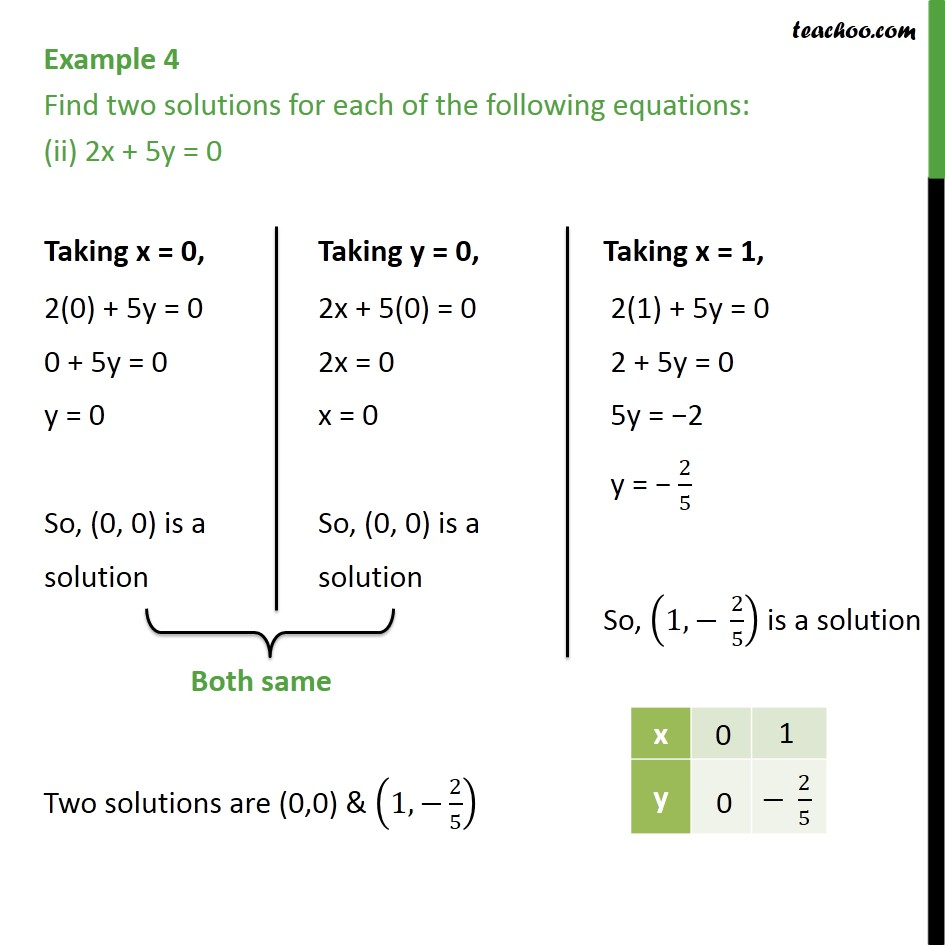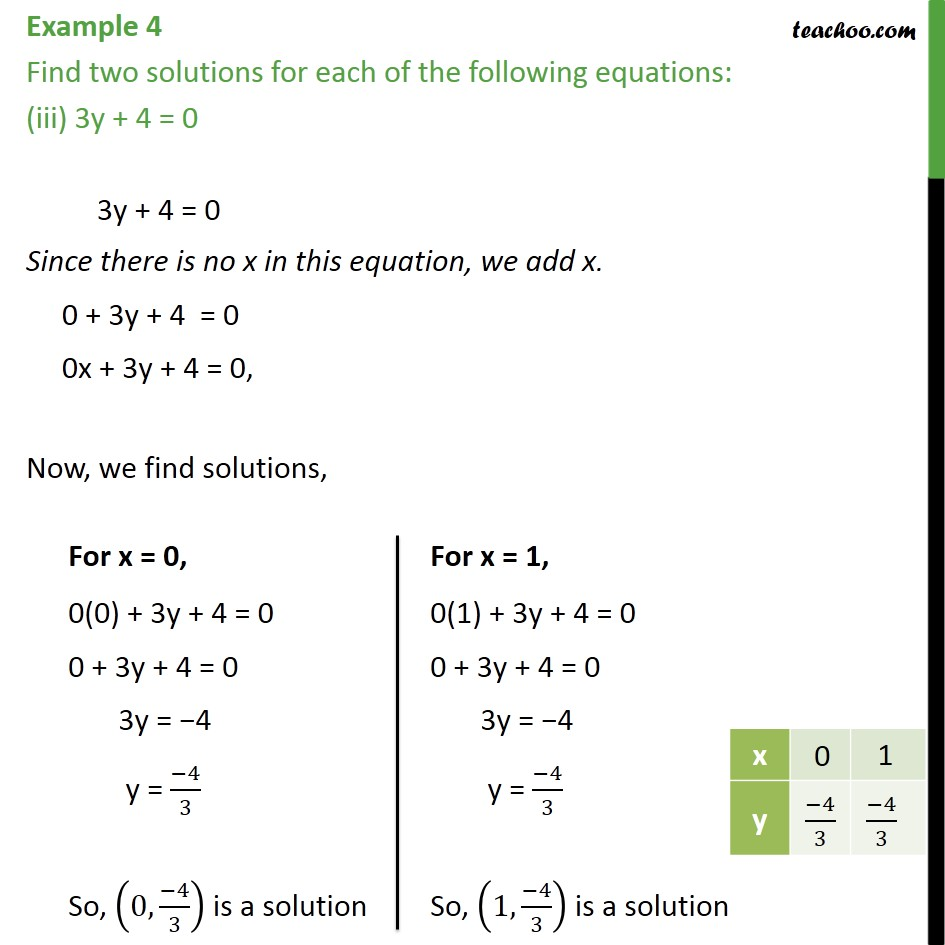Learn all Concepts of Linear Equations Class 9 (with VIDEOS). Check - Linear Equations in 2 Variables Class 91. Chapter 4 Class 9 Linear Equations in Two Variables
2. Serial order wise
3. Examples

Transcript

Example 4 Find two solutions for each of the following equations: 4x + 3y = 12 4x + 3y = 12 Two solutions are (0,4) & (3,0) Example 4 Find two solutions for each of the following equations: (ii) 2x + 5y = 0 Two solutions are (0,0) & (1, 2/5) Example 4 Find two solutions for each of the following equations: (iii) 3y + 4 = 0 3y + 4 = 0 Since there is no x in this equation, we add x. 0 + 3y + 4 = 0 0x + 3y + 4 = 0, Now, we find solutions,

Examples